Test 10 – Question 30

When they tell you that, they’re telling you that angleis also 90°. And that means if you draw one more segment, straight down from point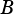to a new point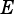on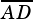, you break the quadrilateral into a rectangle and a right triangle. Like so:

Now, we know in a rectangle, the opposite sides are congruent. That means the new segment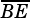we drew has the same length as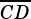. And because the question told us, it’s also true that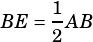.

So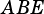is a right triangle, and its hypotenuse is twice as long as one of its legs. That’s a special right triangle! A 30°-60°-90°, to be precise.is the short leg, so angle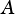will be the 30° angle. That means anglemeasures 60°. We know angle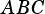is 90°, so anglefrom the original figure measures 150°.